Examples

Chapter 4 Class 8 Data Handling
Serial order wise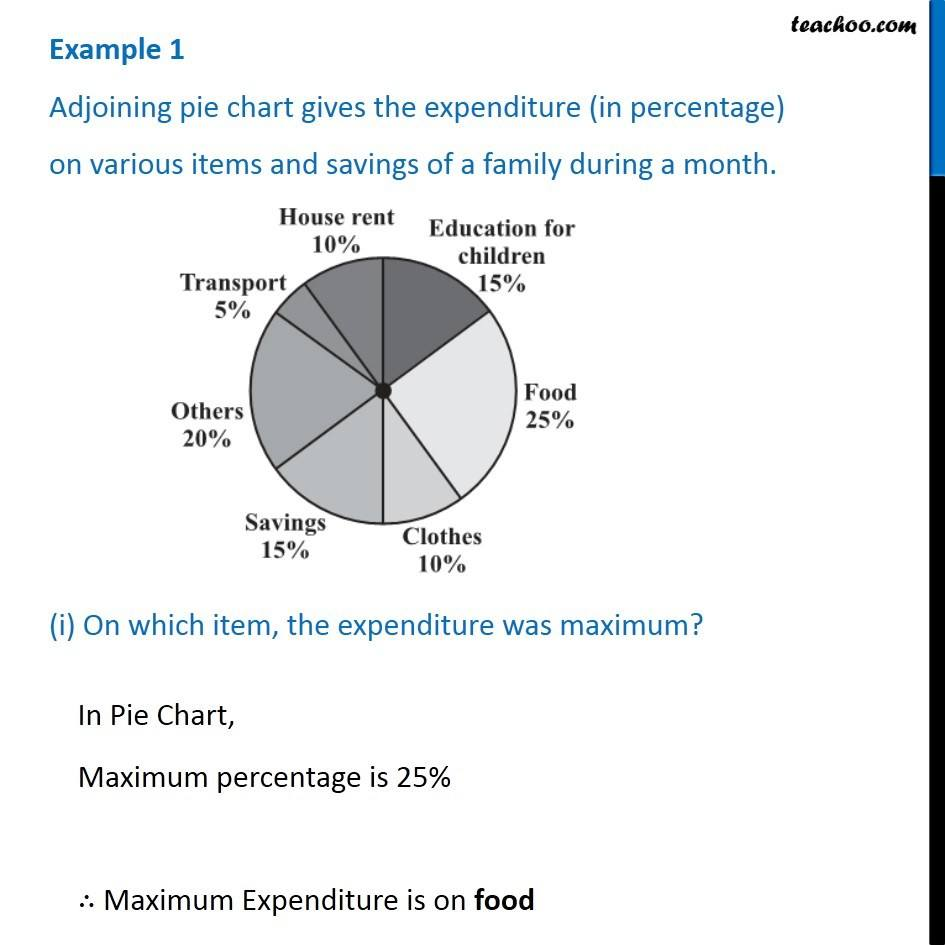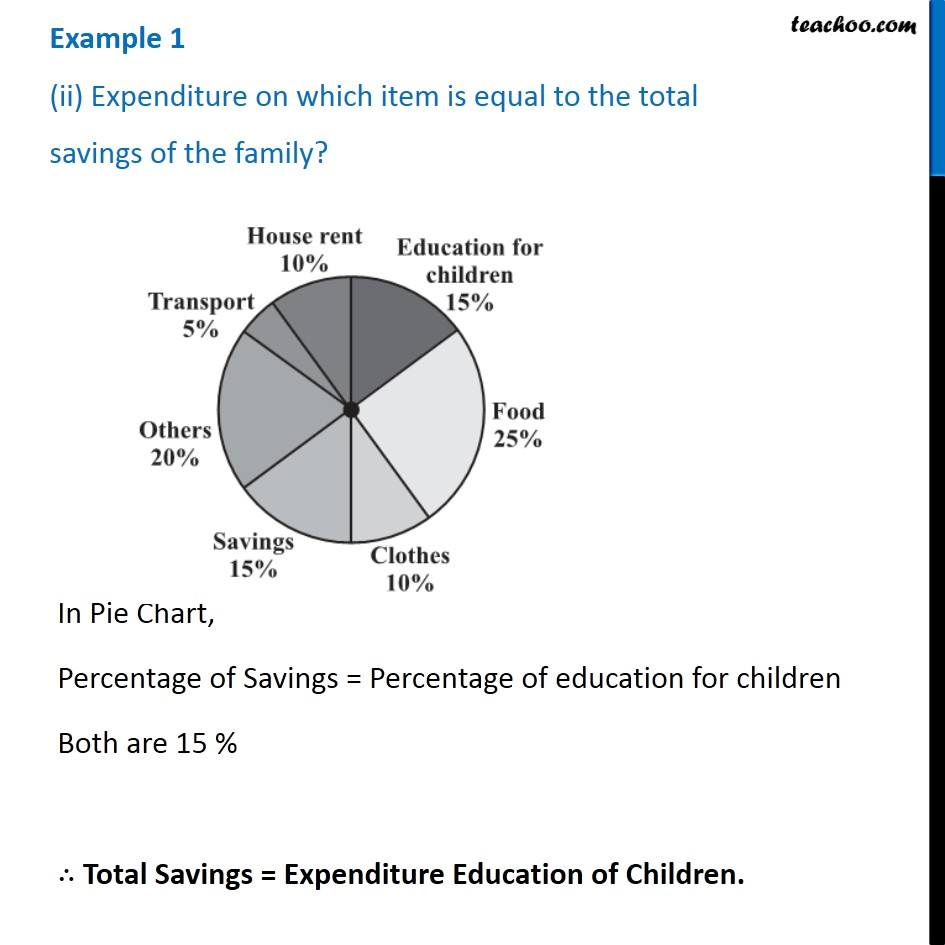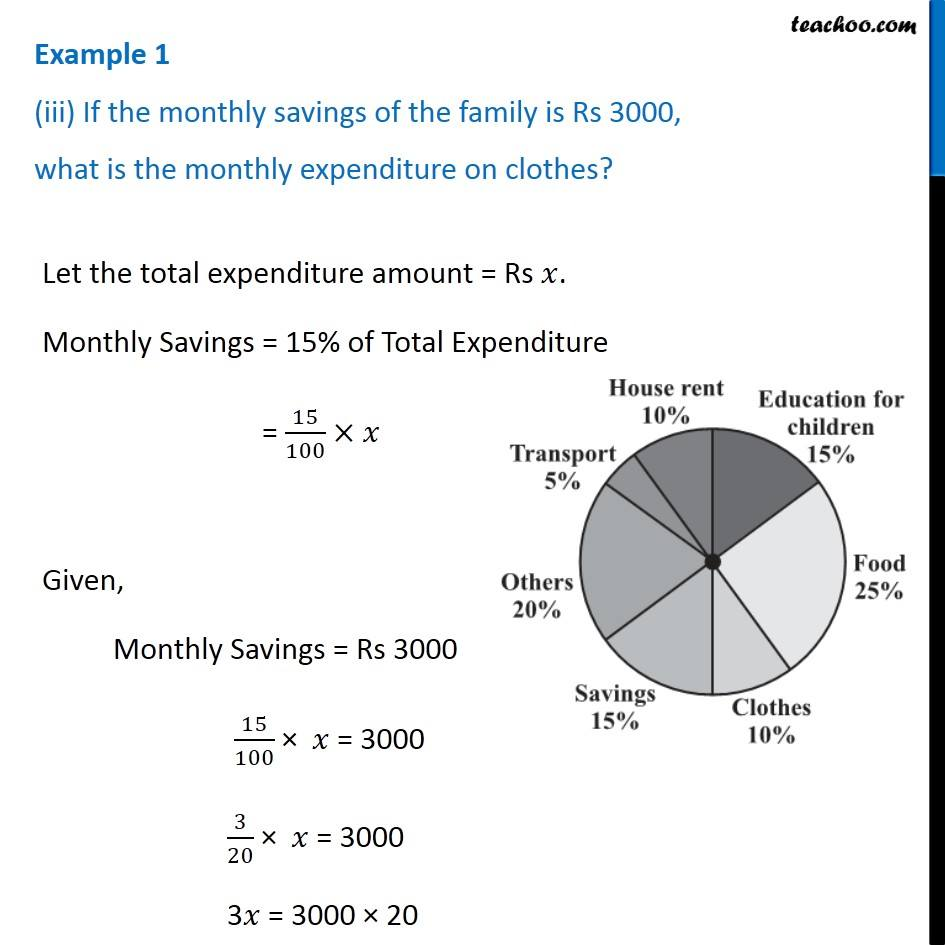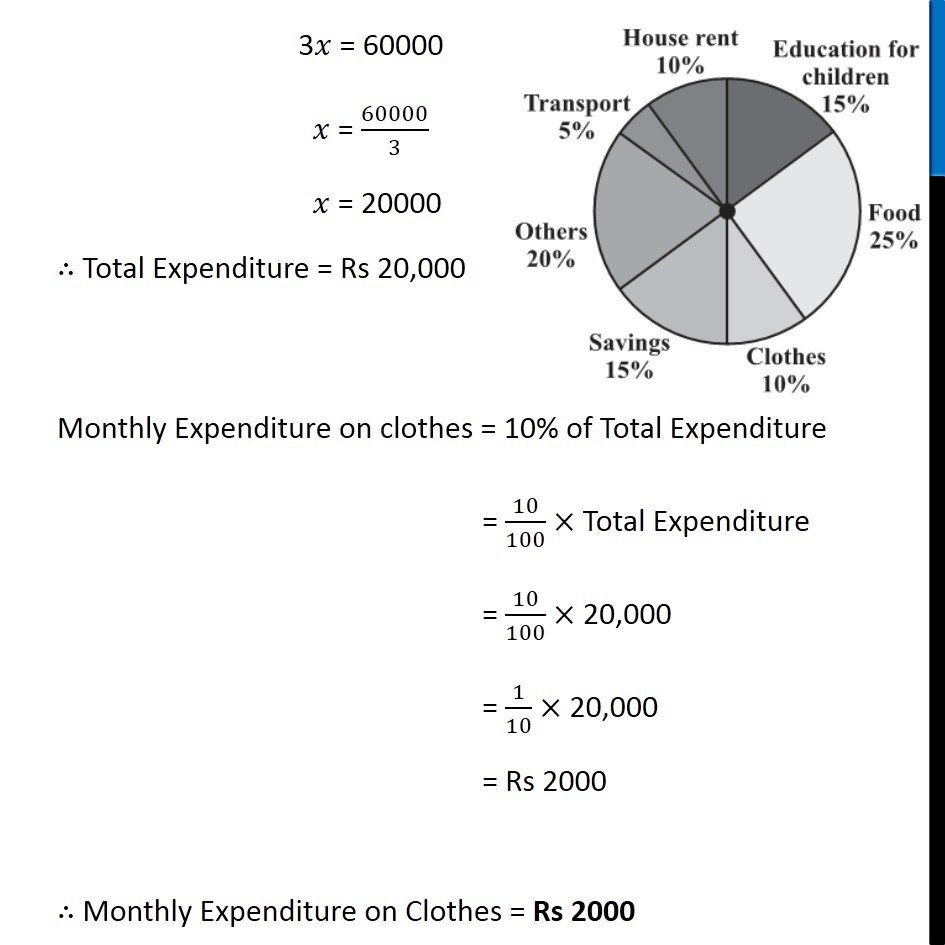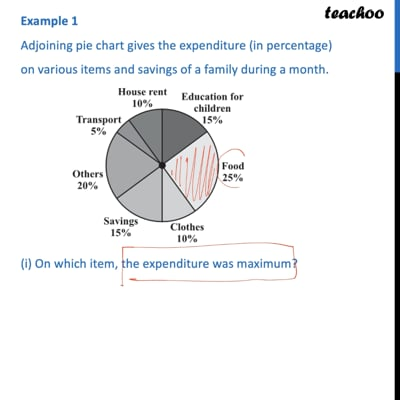This video is only available for Teachoo black users

Learn in your speed, with individual attention - Teachoo Maths 1-on-1 Class

### Transcript

Example 1 Adjoining pie chart gives the expenditure (in percentage) on various items and savings of a family during a month. (i) On which item, the expenditure was maximum?In Pie Chart, Maximum percentage is 25% ∴ Maximum Expenditure is on food In Pie Chart, Maximum percentage is 25% ∴ Maximum Expenditure is on food Example 1 (iii) If the monthly savings of the family is Rs 3000, what is the monthly expenditure on clothes? Let the total expenditure amount = Rs 𝑥. Monthly Savings = 15% of Total Expenditure = 15/100×𝑥 Given, Monthly Savings = Rs 3000 15/100 × 𝑥 = 3000 3/20 × 𝑥 = 3000 3𝑥 = 3000 × 20 3𝑥 = 60000 𝑥 = 60000/3 𝑥 = 20000 ∴ Total Expenditure = Rs 20,000 Monthly Expenditure on clothes = 10% of Total Expenditure = 10/100× Total Expenditure = 10/100× 20,000 = 1/10× 20,000 = Rs 2000 ∴ Monthly Expenditure on Clothes = Rs 2000